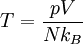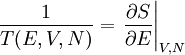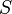Difference between revisions of "Temperature"

The temperature of a system in classical thermodynamics is intimately related to the zeroth law of thermodynamics; two systems having to have the same temperature if they are to be in thermal equilibrium (i.e. there is no net heat flow between them). However, it is most useful to have a temperature scale. By making use of the ideal gas law one can define an absolute temperature$T = \frac{pV}{Nk_B}$

however, perhaps a better definition of temperature is$\frac{1}{T(E,V,N)} = \left. \frac{\partial S}{\partial E}\right\vert_{V,N}$

where$S$ is the entropy.

Units

Temperature has the SI units of kelvin (K) (named in honour of William Thomson) The kelvin is the fraction 1/273.16 of the thermodynamic temperature of the triple point of water.